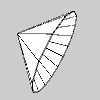# Py5Vector.rotate_around()#

Rotate around an arbitrary 3D vector.

## Examples#```def setup():
py5.size(100, 100, py5.P3D)
py5.translate(10, 30, -20)
v1 = py5.Py5Vector(70, 0, -10)
cone_center = py5.Py5Vector(20, 10, 7)
with py5.begin_shape(py5.TRIANGLE_FAN):
py5.vertex(0, 0, 0)
for i in range(16):
v1.rotate_around(-py5.TWO_PI / 15, cone_center)
py5.vertex(v1.x, v1.y, v1.z)
```

## Description#

Rotate around an arbitrary 3D vector. This method is only applicable to 3D vectors. Use the `angle` parameter to specify the rotation angle and the `v` parameter to specify the vector to rotate around. The `v` vector does not need to be aligned to any axis or normalized, but it must be a 3D vector and it cannot be a vector of zeros.

The vector’s rotation will follow the right-hand rule. Using your right hand, point your thumb in the direction of the vector to rotate around. Your fingers will curl in the direction of rotation when the `angle` parameter is positive.

## Signatures#

```rotate_around(
angle: float,  # angle of rotation, measured in radians
v: Py5Vector3D,  # 3D vector to rotate vector around
) -> Py5Vector3D
```

Updated on September 01, 2022 16:36:02pm UTC